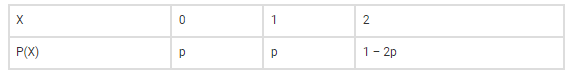# The random variable X can take only the values 0, 1, 2.`
Question:

The random variable X can take only the values 0, 1, 2. Given that P(X = 0) = P (X = 1) = and that E(X2) = E[X], find the value of p.

Solution:

Given, X = 0, 1, 2 and P(X = 0) = P (X = 1) = p

Let P(X) at X = 2 is x

⇒ p + p + x = 1 ⇒ x = 1 – 2p

Now, we have the following distribution:So,

E(X) = 0.p + 1.p + 2(1 – 2p) = p + 2 – 4p = 2 – 3p

And, E(X2) = 0.p + 1.p + 4(1 – 2p) = p + 4 – 8p = 4 – 7p

Also, given E(X) = E(X2)

2 – 3p = 4 – 7p

4p = 2

p = ½

Therefore, the required value of p is ½.